# Matrix (mathematics) facts for kids

Kids Encyclopedia Facts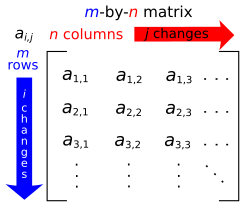Specific entries of a matrix are often referenced by using pairs of subscripts, for the numbers at each of the rows & columns.

In mathematics, a matrix (plural: matrices) is a rectangle of numbers, arranged in rows and columns. The rows are each left-to-right (horizontal) lines, and the columns go top-to-bottom (vertical). The top-left cell is at row 1, column 1 (see diagram at right).

Matrices are often represented by capital roman letters such as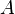$A$,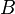$B$ and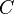$C$, and there are rules for adding, subtracting and "multiplying" matrices together, but the rules are different than for numbers. As an example, the product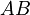$AB$ does not always give the same result as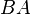$BA$, which is the case for the multiplication of ordinary numbers. A matrix can have more than 2 dimensions, such as a 3D matrix. Also, a matrix can be one-dimensional, as a single row or a single column.

Many natural sciences use matrices quite a lot. In many universities, courses about matrices (usually called linear algebra) are taught very early, sometimes even in the first year of studies. Matrices are also very common in computer science, engineering, physics, economics, and statistics.

## Definitions and notations

The horizontal lines in a matrix are called rows and the vertical lines are called columns. A matrix with m rows and n columns is called an m-by-n matrix (or m×n matrix) and m and n are called its dimensions.

The places in the matrix where the numbers are is called entries. The entry of a matrix A that lies in the row number i and column number j is called the i,j entry of A. This is written as A[i,j] or ai,j.

We write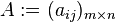$A:=(a_{ij})_{m \times n}$ to define an m × n matrix A, with each entry in the matrix called ai,j for all 1 ≤ im and 1 ≤ jn.

### Example

The matrix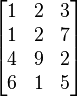$\begin{bmatrix} 1 & 2 & 3 \\ 1 & 2 & 7 \\ 4 & 9 & 2 \\ 6 & 1 & 5 \end{bmatrix}$

is a 4×3 matrix. This matrix has m=4 rows, and n=3 columns.

The element A[2,3] or a2,3 is 7.

## Operations

The sum of two matrices is the matrix, which (i,j)-th entry is equal to the sum of the (i,j)-th entries of two matrices: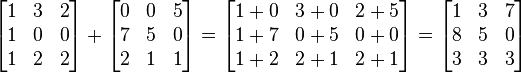$\begin{bmatrix} 1 & 3 & 2 \\ 1 & 0 & 0 \\ 1 & 2 & 2 \end{bmatrix} + \begin{bmatrix} 0 & 0 & 5 \\ 7 & 5 & 0 \\ 2 & 1 & 1 \end{bmatrix} = \begin{bmatrix} 1+0 & 3+0 & 2+5 \\ 1+7 & 0+5 & 0+0 \\ 1+2 & 2+1 & 2+1 \end{bmatrix} = \begin{bmatrix} 1 & 3 & 7 \\ 8 & 5 & 0 \\ 3 & 3 & 3 \end{bmatrix}$

The two matrices have the same dimensions. Here,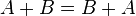$A + B = B + A$ is true (and is true in general for matrices of equal dimensions).

### Multiplication of two matrices

The multiplication of two matrices is a bit more complicated:

$bmatrix} a1 & a2 \\ a3 & a4 \\ \end{bmatrix} \cdot \begin{bmatrix} b1 & b2 \\ b3 & b4 \\ \end{bmatrix} = \begin{bmatrix} (a1\cdot b1 + a2 \cdot b3) & (a1 \cdot b2 + a2 \cdot b4) \\ (a3\cdot b1 + a4 \cdot b3) & (a3 \cdot b2 + a4 \cdot b4) \\ \end{bmatrix$

So with numbers: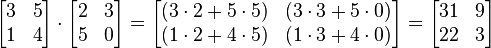$\begin{bmatrix} 3 & 5 \\ 1 & 4 \\ \end{bmatrix} \cdot \begin{bmatrix} 2 & 3 \\ 5 & 0 \\ \end{bmatrix} = \begin{bmatrix} (3\cdot 2 + 5 \cdot 5) & (3 \cdot 3 + 5 \cdot 0) \\ (1\cdot 2 + 4 \cdot 5) & (1 \cdot 3 + 4 \cdot 0) \\ \end{bmatrix} = \begin{bmatrix} 31 & 9 \\ 22 & 3 \\ \end{bmatrix}$
• Two matrices can be multiplied with each other even if they have different dimensions, as long as the number of columns in the first matrix is equal to the number of rows in the second matrix.
• The result of the multiplication, called the product, is another matrix with the same number of rows as the first matrix and the same number of columns as the second matrix.
• The multiplication of matrices is not commutative, which means that in general,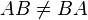$AB \neq BA$.
• The multiplication of matrices is associative, which means that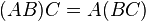$(AB)C = A(BC)$.

## Special matrices

There are some matrices that are special.

### Square matrix

A square matrix has the same number of rows as columns, so m=n.

An example of a square matrix is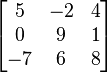$\begin{bmatrix} 5 & -2 & 4 \\ 0 & 9 & 1 \\ -7 & 6 & 8 \\ \end{bmatrix}$

This matrix has 3 rows and 3 columns: m=n=3.

### Identity

Every square dimension set of a matrix has a special counterpart called the "identity matrix", represented by the symbol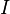$I$. The identity matrix has nothing but zeroes except on the main diagonal, where there are all ones. For example:$\begin{bmatrix} 1 & 0 & 0 \\ 0 & 1 & 0 \\ 0 & 0 & 1 \\ \end{bmatrix}$

is an identity matrix. There is exactly one identity matrix for each square dimension set. An identity matrix is special because when multiplying any matrix by the identity matrix, the result is always the original matrix with no change.

### Inverse matrix

An inverse matrix is a matrix that, when multiplied by another matrix, equals the identity matrix. For example: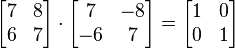$\begin{bmatrix} 7 & 8 \\ 6 & 7 \\ \end{bmatrix} \cdot \begin{bmatrix} 7 & -8 \\ -6 & 7\\ \end{bmatrix} = \begin{bmatrix} 1 & 0 \\ 0 & 1 \\ \end{bmatrix}$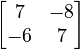$\begin{bmatrix} 7 & -8 \\ -6 & 7 \\ \end{bmatrix}$ is the inverse of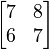$\begin{bmatrix} 7 & 8 \\ 6 & 7 \\ \end{bmatrix}$.

The formula for the inverse of a 2x2 matrix,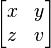$\begin{bmatrix} x & y \\ z & v \end{bmatrix}$ is: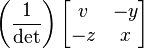$\left ( \frac{1}{\det} \right )\begin{bmatrix} v & -y \\ -z &x \end{bmatrix}$

Where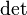$\det$ is the determinant of the matrix. In a 2x2 matrix, the determinant is equal to: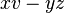${xv-yz}$

### One column matrix

A matrix, that has many rows, but only one column, is called a column vector.

## Determinants

The determinant takes a square matrix and calculates a simple number, a scalar. To understand what this number means, take each column of the matrix and draw it as a vector. The parallelogram drawn by those vectors has an area, which is the determinant. For all 2x2 matrices, the formula is very simple: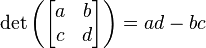$\det\left( \begin{bmatrix} a & b \\ c & d \\ \end{bmatrix}\right) =ad - bc$

For 3x3 matrices the formula is more complicated:$\det\left( \begin{bmatrix} a_1 & b_1 & c_1 \\ a_2 & b_2 & c_2 \\ a_3 & b_3 & c_3 \\ \end{bmatrix}\right) = a_1(b_2 c_3 - c_2 b_3) - a_2(b_1 c_3 - c_1 b_3) + a_3(b_1 c_2 - c_1 b_2)$

There are no simple formulas for the determinants of larger matrices, and many computer programmers study how to get computers to quickly find large determinants.

### Properties of determinants

There are three rules that all determinants follow. These are:

• The determinant of an identity matrix is 1
• If two rows or two columns of the matrix are exchanged, then the determinant is multiplied by -1. Mathematicians call this alternating.
• If all the numbers in one row or column are multiplied by another number n, then the determinant is multiplied by n. Also, if a matrix M has a column v that is the sum of two column matrices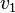$v_1$ and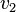$v_2$, then the determinant of M is the sum of the determinants of M with$v_1$ in place of v and M with$v_2$ in place of v. These two conditions are called multi-linearity.

## Images for kidsMatrix (mathematics) Facts for Kids. Kiddle Encyclopedia.